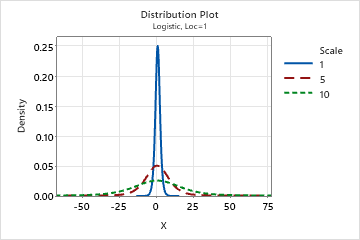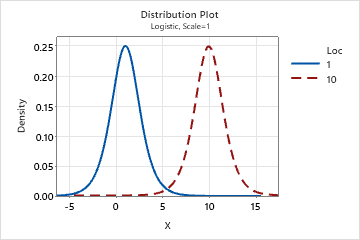# Logistic distribution

Use the logistic distribution to model data distributions that have longer tails and higher kurtosis than the normal distribution.

The logistic distribution is a continuous distribution that is defined by its scale and location parameters. The logistic distribution has no shape parameter, which means that the probability density function has only one shape. The shape of the logistic distribution is similar to that of the normal distribution. However, the logistic distribution has longer tails.
Effect of scale parameter
The following graph shows the effect of the different values of the scale parameter on the logistic distribution.Effect of location parameter
The following graph shows the effect of the different values of the location parameter on the logistic distribution.By using this site you agree to the use of cookies for analytics and personalized content.  Read our policy